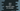# Java program to find the absolute value of a number## How to find the absolute value of a number in Java:

To find the absolute value of a number, we can use Math.abs method. This is a static method and we can use it with double, float, int or long. In this post, we will learn how to use Math.abs method with example.

### Definition of Math.abs:

This method is defined as below:

``public static dtype abs(dtype number)``

The dtype is the data type and it can be a float, double, integer or long. It returns the absolute value in same data type.

### Example of Math.abs:

Below example shows how Math.abs works with different parameters:

``````public class Example {
public static void main(String[] args) {
System.out.println(Math.abs(-29));
System.out.println(Math.abs(-29.9));
System.out.println(Math.abs(-20.5434));
}
}``````

It will print the below output:

``````29
29.9
20.5434``````

Similarly, we can use Math.abs with float and long values.

``````public class Example {
public static void main(String[] args) {
float value1 = -5.67f;
long value2 = -654L;

System.out.println(Math.abs(value1));
System.out.println(Math.abs(value2));
}
}``````

It will print:

``````5.67
654``````